#### Motion in Central Force Fields, Physics tutorial

Vector Analysis:

Physics makes use of equations, formulas, and vectors. The vector is the special kind of quantity in which there are two components: magnitude and direction. Vectors are utilized commonly in physics to represent phenomena like force, velocity, and acceleration. The simple example is motion of the airplane. Speed of airplane combined with the direction of motion together constitutes the vector quantity known as velocity. In contrast, real numbers, also known as scalars, are one-dimensional (they can be depicted on the line); they have only magnitude. Scalars are satisfactory for signifying phenomena or quantities like temperature, time, and mass.

Vector Position: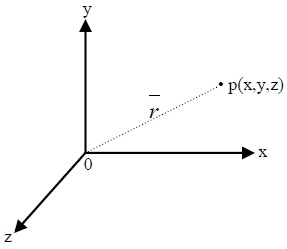Point P is in Cartesian coordinates and has no direction; such quantity is known as scalar. Though, op‾ is the position vector that defines position of point p from origin.

op‾ = r‾(position vector) = f(x, y, z)

= i^x j^y + K^z

The letters i^, j^, k^ (could also be written in bold i, j, k) are called 'unit vectors' and

r2 = x2 + y2 + z2

Unit vector = r‾/|r|

When point p coincides with origin 0, r‾ 0 (zero), this particular vector op‾ = 0 is known as 'zero vector'

Gradient, Divergence and Curl:

Fields are quantities which are function of position in space.

There are two kinds of fields: scalar field, denoted Φ(x, y, z) or Φ(r‾) and vector field, denoted A‾(x, y, z) or A‾(r‾). Both are functions of position in space.

Define a symbol

∇ = del = i^ ∂/∂x + j^∂/∂y + k^∂/∂z

(i) ∇Φ = (delΦ) = grad(Φ) ≡ gradient of the scalar field Φ

i^∂/∂x + j^∂/∂y + k^∂/∂z ≡ gradient of the scalar gives the vector

Divergence of vector A‾(can also be A^, A→ etc) is

∇.A‾ = DivA‾ (divergence of A‾)

= (i^∂/∂x + j^∂/∂y + k^∂/∂z).(i^Ax + j^Ay + k^Az)

= ∂Ax/∂x + ∂Ay/∂y + ∂Az/∂z ≡ gives scalar quantity

∇xA‾ = CurlA‾ = (i^∂/∂x + j^∂/∂y + k^∂/∂z)x(i^Ax + j^Ay + k^Az)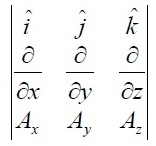Central - Conservative Forces:

The central force is the conservative field, i.e., it can always be stated as negative gradient of the potential: The force which can be derived from the potential energy V(r) which only depends on distance to source is known as central. As a result of being conservative, the central force field is irrotational, i.e., its curl is zero. As central force is conservative energy of the planet should be a constant over orbit. Gravitational force, electric force between charges and spring force in elevator are examples of central forces. An external force is said to be central if it is always directed towards or away from a fixed point, called 'the centre of force'.

If we select the origin to be at this centre, this signifies that F‾ is always parallel to r‾.

Mathematically,

F‾ = f(r‾)‾r1

Where r1‾ = r‾/|r| ≡ unit vector

If f(r‾)<0 is attractive

f(r‾)> 0 is repulsive

Properties of Central Force Fields:

If a particle moves under action of the central force field;

(a) Angular momentum of particle is conserved

(b) Path (or orbit ) of particle should be the plane curve.

I.e. Particle moves in the plane curve

proof:

Central force field

F‾ = f(r)r1‾ where r1‾ is unit vector

r‾x F‾ = r‾x f(r)r‾ where r1‾ is unit vector

r‾ x F‾ = r‾x f(r)r1‾= 0, F = ma‾ = mdv‾/dt

r‾ x F‾ = r‾xmdv‾dt = 0 (since r‾ x F‾ = 0)

So md(r‾x v‾ = 0)/dt = 0

r‾xv‾ = constant (h, say)

r‾.(r‾xv‾) = r‾.h‾

0 . v‾ = r‾.h‾ (as r‾ x r‾ = 0 )

0 = r‾. h‾ = r is perpendicular to h‾ (i.e r - h‾ )

Work Performed by Conservative force fields:

From everyday experience, work is associated with magnitude of force acting during performing the work and magnitude of the displacement of body from one point to another. Generally, work does depend on path taken between any two points.

Let V be a scalar function such that

F‾ = -∇V where F‾ = ma ≡ force field ( a vector space)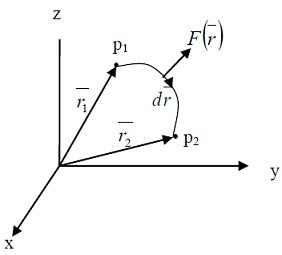We want to find the work W, done by the force F in moving a particle from point p1 to p2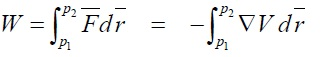Moving particle from point p1 to p2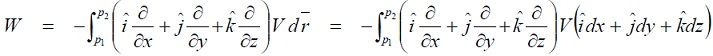W = -∫p1p2dv = [-V]p1p2 = V(p1) - V(p2) = V1  - V2

V1 and V2 are potential energies (or simply potentials) at point p1 and p2 respectively. The work done is independent of path transversed by particle. If work done by the force field in moving the particle from one point to another point is independent of path joining the points, then force field is said to be conservative.

Precondition:

F‾ = -∇V

∇‾x F‾ = ∇x(-∇V)=0

• It can be demonstrated that force field F(r) is conservative implies that ?F(r).dr = 0
• ?F(r).dr = 0 for any closed path signifies 'there exists function V(r) such that F(r) = -∇V(r)
• F‾ is conservative if curl F = ∇‾ x F‾ = 0

Definition of Central Conservative Forces:

These are forces that show both properties of conservative and central forces. Central forces are always conservative.

Conservative theorems:

Energy:

Work done W by net force on the particle by displacing it from the initial potential V1 to a final potential V2 equals change in particle's kinetic energy from T1 to T2.

W = V1 - V2

W = T2 - T1

Equating two equations,

V1 - V2 = T2 - T1

i.e. V1 + T1 = V2 + T2 = constant

T + V = K.E + P.E = E (total energy)

This result is called as work - energy theorem.

Torque and Conservation of Angular momentum:

When net external torque acting on system is zero, total angular momentum of system is constant (conserved).  Torque, N is moment of the force.

N‾ = r‾ x F‾ = r x d/dt(mv‾) = d/dt(r‾ x mv‾) using vector identity

= d/dt(r‾ x p‾)

r‾ x p‾ = L‾ (angular momentum)

N‾ = dL‾/dt

If N‾ = 0

dL‾/dt = 0

= L‾ = constant (implies L is conserved)

N‾ = r‾ x F‾ = dL‾/dt

Here r‾ is parallel to F‾

=r‾ x F = 0 i.e. N‾ = 0

dL‾/dt = 0 L‾ = constant (means is conserved)

Impulse and Conservation of Linear momentum, p:

Change in momentum of the particle during a time interval equals the impulse of the net force which applies on particle during that interval.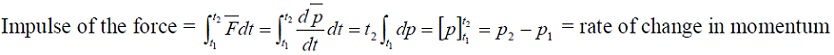F‾ = dp‾/dt

If F‾ = 0

dp‾/dt = 0

p‾ = = constant (implies p is conserved)

This result is called as impulse - momentum theorem

Kinematics in Polar coordinates:

Mechanics frequently comprises study of the motion of particles along curves; this is called as kinematics. In problems with particular symmetries, it is frequently suitable to use non- Cartesian co-ordinates. In particular, in case of axial or spherical symmetry, we may utilize cylindrical polar co-ordinates ρ, ?, z, or spherical polar co-ordinates r, Θ, ?. These co-ordinates, though curvilinear, are still orthogonal in sense that three coordinate directions at each point are mutually perpendicular. These are associated to Cartesian co-ordinates.

Velocity and acceleration components in polar coordinates:

In several practical problems, potential energy function is spherically symmetric; it relies only on distance from origin

r^=etΘ^+etr^

This is the particles velocity, acceleration is given by:

= 2etΘ^

Energy Conservation in Central - Conservative Force Fields:

For a particle moving under any central, conservative force, information about the radial motion may be obtained from the radial energy equation., which results from eliminating the time derivative of θ with respect to time from equations of a particle motion in central conservative force field.

1/2mr2 + L2/2mr2 + V(r) = E

Energy Conservation Equation:

For a particle which moves in a central force field described by a potential function, it possesses spherical symmetry and angular momentum and total energy are conserved.

(du/dΘ)2 + u2 = 2m/L2(E - V)

This equation can be integrated to obtain the equation of the orbit in any of the following the forms

r = r(Θ)

r = r(t)

Θ = Θ(t)

Equation of Orbit:

If eliminate time from the equations of motion, we get the path of the particle.

m(-h2u2d2udΘ - h2u3) = f(1/u) or d2u/dΘ2 + u = -f(1/u)/(mh2u2)

This equation can be applied to determine path of particle that force involved is directed towards the centre (i.e. ellipse, circular, parabolic, hyperbolic, etc.).

For the given central force, increasing velocity causes the orbit to change from the circle to ellipse to a parabola to a hyperbola, with changes happening at certain critical velocities.

Central - Conservative Force and Planetary Motion:

When satellite moves in circular orbit, centripetal acceleration is given by gravitational attraction of earth. Kepler's laws explain more general case: elliptical orbit of the planet around sun or the satellite around the planet

Kepler's laws will be reviewed and orbits of the planet surrounding the heavy sun described. Differential equation for orbit of motion in the inverse square law force field is derived.

Kepler's Laws:

Kepler's three laws of planetary motion can be explained as follows:

Paths of planets about sun are elliptical in shape, with center of the sun being located at one focus. (The Law of Ellipses)

The imaginary line drawn from center of the sun to the center of the planet will sweep out equal areas in equal intervals of time. (The Law of Equal Areas)

Ratio of squares of periods of any two planets is equal to ratio of cubes of their average distances from sun. (The Law of Harmonies)

Of course, Kepler's Laws originated from observations of the solar system. It must be known that these laws are results of Newton's laws of gravitation and of Motion.

Motion in an Inverse Square Law Force Field:

Thus differential equation for orbit of motion in an inverse square law force field is

d2u/dΘ2 + u = k/mh2

Given that a particle is continuously subjected to the force directed toward the fixed central point, and that magnitude of the force is inversely proportional to square of the particle's distance from that central point, normal way of finding motion of the particle is by solving suitable differential equation. It can easily be shown that particle's path will be the conic, that is, an ellipse, hyperbola, or parabola, with central point located at one focus, and that line from central point to particle sweeps out equal areas in equal times.

Tutorsglobe: A way to secure high grade in your curriculum (Online Tutoring)

Expand your confidence, grow study skills and improve your grades.

Since 2009, Tutorsglobe has proactively helped millions of students to get better grades in school, college or university and score well in competitive tests with live, one-on-one online tutoring.

Using an advanced developed tutoring system providing little or no wait time, the students are connected on-demand with a tutor at www.tutorsglobe.com. Students work one-on-one, in real-time with a tutor, communicating and studying using a virtual whiteboard technology.  Scientific and mathematical notation, symbols, geometric figures, graphing and freehand drawing can be rendered quickly and easily in the advanced whiteboard.

Free to know our price and packages for online physics tutoring. Chat with us or submit request at info@tutorsglobe.com